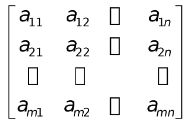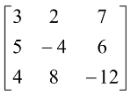# Definition of a Matrix

## Matrices and Determinants of Class 12

A set of mn numbers (real or complex) arranged in the form of a rectangular array having 'm' rows & 'n' columns is called an m × n matrix. [to be read as m by n matrix].

It is usually written as

A =It is represented as A = [aij]m × n

The numbers a11, a12… etc are called elements of the matrix. The element aij belongs to ith row and jth column.

e.g. A =is a 3 × 3 matrix.